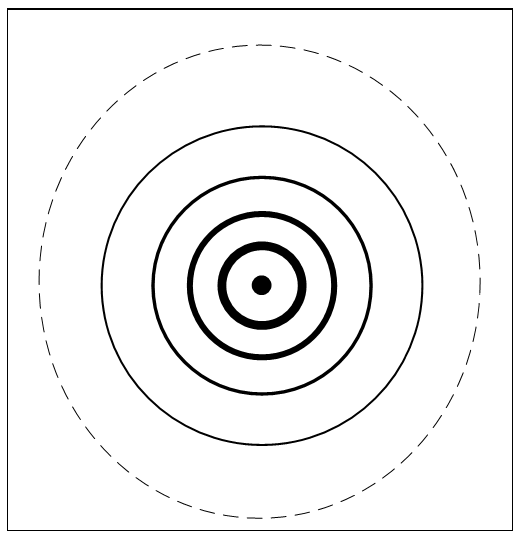# QR3.4.1 Light Spreads

In quantum realism, any processing put on the grid immediately spreads out in all directions, like ripples on a pool but in three dimensions. Not only is a photon’s processing shared among the nodes of its wavelength, it is also passed on in every direction at one node per cycle, i.e. the speed of light. Huygen’s principle, that light is a wave spreading with each point a new wave source, then works because quantum processing spreads. A ray of light isn’t just going forward on a linear path but spreading out in all directions at the same time, hence it goes through both Young’s slits at once.Figure 3.12. Pond ripples spread

Gauss noted that when a pebble drops in a pool, the initial energy spreads out in ripples of decreasing strength such that the energy flux per ripple is constant, but for friction. As shown in Figure 3.12, each ripple is the same energy just spread out over a larger circle. The same principle applied to the three-dimensional “ripple” of light is that the processing flux is constant and there is no friction. This quantum “flux” spreading on a sphere surface reduces power as an inverse square of distance, giving a basis for the inverse square laws of electrical, magnetic and gravitational fields. In addition, processing values cancel at the node as fields do at a point and processing is passed on every cycle at the speed of light, just as fields propagate. The next chapter attributes all the fields of the standard model to one quantum field.

Next